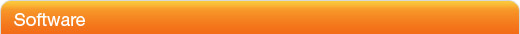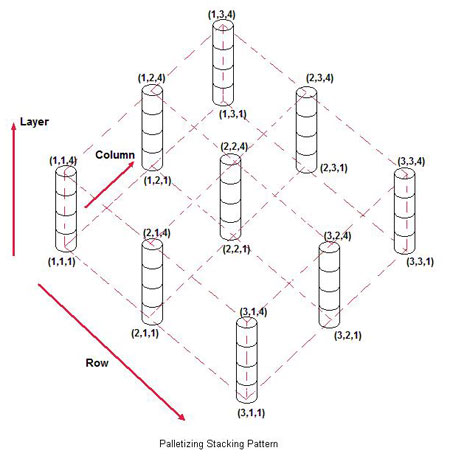# Palletizing Operations

One of the more popular applications for robots is palletizing, which is accomplished by combining a series of commands into a Palletizing routine. A palletizing routine consists of five program instructions, or lines. The first instruction is the Palletize instruction, which is followed by a linear motion instruction to move to the Stack point. The stack point is the top-center of the workpiece, and a group of stack points forms a stacking pattern. The first stack point in a stacking pattern is located at (1,1,1) and is incremented each time the palletizing routine is executed.The number of workpieces to be stacked in a palletizing routine is a product of the number of rows, columns, and layers. In equation form:

# of workpieces palletized = RCL

where      R = # of rows
C = # of columns
L = # of layers

The first command in a palletizing routine determines the approach/retract point. It does this for each workpiece to be stacked every time the routine executes. As part of this first command, the robot moves the workpiece to the approach/retract point. The second command moves the workpiece in a linear (downward) direction to the stack point. The third command opens the gripper. The fourth command is a WAIT instruction, and the fifth is the PAL_END instruction. The PAL_END instruction moves the end effector directly upward to the approach/retract point.

In order to perform a palletizing operation, the RCL values are set using the PAL instruction, and a counting routine is required to count the number of boxes to be stacked and to stop the robot when the count is complete. The count is generally accomplished in palletizing operations by using an IF instruction to increment the count and an IF instruction to terminate the count when the preset value equals the actual value.

The following IF instruction can be used to automatically increment a register by a value of 1:

R = R + R       where, R = 1

With this instruction, R is initially zero and each time the instruction is executed the value of R increases by a value of 1.

The following IF instruction can be used to compare the value of the incremented register with the preset value to be counted:

IF R <= R THEN
JMP_LBL 1

With this instruction, if R is less than or equal to R then the program is instructed to jump to the beginning of the program routine. If R is greater than R, then the program will stop.

The following program is an example of a palletizing routine to stack 18 workpieces on a pallet:

 Program code Conveyor 1 FWD WAIT 20 seconds LBL 1 R = R + R Conveyor 1 FWD AP 100 deg/sec LP  100 cm/sec WAIT 4 seconds GRIPPER Close LP  100 cm/sec Conveyor 1 FWD AP 100 deg/sec PALLETIZE ( 3 3 2) LP  100 cm/sec GRIPPER Open WAIT 4 seconds PAL _END IF R <= R THEN JMP_LBL 1 Comment Start Conveyor 1 Load workpiece Start palletizing routine Increment register instruction Restart Conveyor 1 Move to 1st position point Move to 2nd (grip) position point Wait for workpiece to settle Pick up workpiece Retract to 1st position point Restart Conveyor 1 Move towards pallet (3rd pos. point) Set palletize parameters Move closer to pallet (4th pos. point) Release workpiece Wait for workpiece to settle Close palletize parameters Compare contents of two registers Jump to LBL 1

The PALLETIZE command sets the palletizing routine parameters, which in this case are 3, 3, 2. Position Register P has been pre-loaded with the first stacking point (1, 1, 1). Register R[0} has been pre-loaded with the number 0, and register R has been loaded with the number 1. R is the reference, or starting number for the palletize count operation, while register R is the amount that the count increments each time the command is executed. Register R contains the number of workpieces to be stacked by the palletizing operation. In this example, R has been preloaded with the number 18.

Registers R and R are used by the IF instruction R = R + R. This instruction adds the contents of register R to the contents of register R and stores the result in register R. Since register R contains the number 1, each time the loop executes register R increases by 1.

The second IF command, IF R <= R THEN, is used to compare the contents of the incremented register R with the preset value in register R. If the preset value is greater than or equal to the current count, the JMP_LBL 1 command is executed and the program jumps to the beginning of the programmed routine. If the incremented value should try to exceed the preset value, the program will not loop but will instead come to an end.